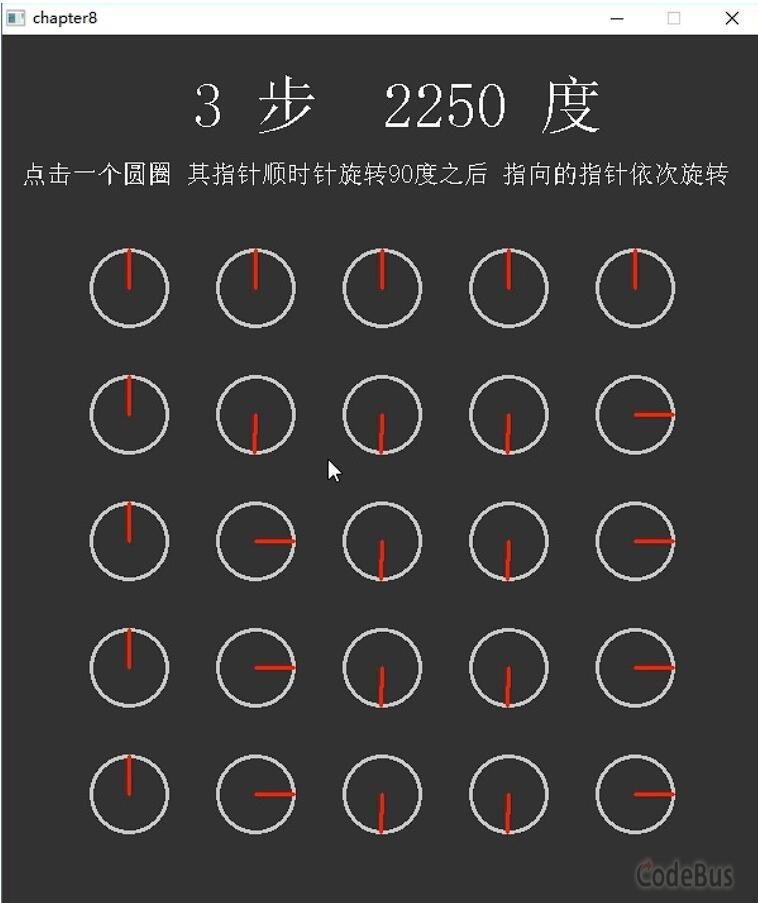# 童晶``````#include <graphics.h>
#include <conio.h>
#include <math.h>
#define PI 3.14159 // PI宏定义

struct Round // 定义结构体，用来表示带角度指示的小圆圈
{
float x,y; // 小圆的圆心坐标
float r; // 小圆半径
int angleNum;  // 对应的角度，这里只能是0,1,2,3。表示乘以 PI/2 后对应的四个角度值
};
// 全局变量定义
Round rounds; // 结构体二维数组
int step; // 还剩下的操作步数
int score; // 得分，也就是一共旋转了多少度

void rotateRound(int i,int j) // 对二维数组中i行j列的小圆圈，顺时针旋转
{
rounds[i][j].angleNum -= 1; // 值减1
if (rounds[i][j].angleNum<0) // 如果小于0，再设为3
rounds[i][j].angleNum = 3;
score += 90; // 得分加上90度
}

// 获得当前圆指向的下一个圆圈序号
// 当前圆序号存储在数组int indexes中，下一个圆序号也存储在这个数组中
// 如果有下一个指向的圆，返回1；如果指向边界了，返回0
int GetNextIndexes(int indexes)
{
int i = indexes; // 当前圆的i，j序号
int j = indexes; //

// 根据当前圆的角度，获得下一个小圆圈的序号
if (rounds[i][j].angleNum==0) // 指向右边的小圆圈
j++; // right
else if (rounds[i][j].angleNum==3) // 指向下边的小圆圈
i++; // down
else if (rounds[i][j].angleNum==2) // 指向左边的小圆圈
j--; // left
else if (rounds[i][j].angleNum==1) // 指向上边的小圆圈
i--; // up

indexes = i; // 在数组中更新指向的下一个圆圈的序号
indexes = j; //

if (i>=0 && i<5 && j>=0  && j<5) // 如果序号没有越界
return 1; // 说明指向了一个圆圈，返回1
else //
return 0; // 没有指向有效圆圈，返回0
}

void startup()  // 初始化函数
{
initgraph(600,700); // 新建画面
setbkcolor(RGB(50,50,50)); // 设置背景颜色
setlinestyle(PS_SOLID,3); //  设置线条样式、线宽
cleardevice(); // 清空背景
BeginBatchDraw(); // 开始批量绘制
step = 10; // 一共可以操作10步
score = 0; // 初始为0度
int i,j;
// 初始化 设定5*5共25个小圆圈
for (i=0;i<5;i++)
{
for (j=0;j<5;j++)
{
rounds[i][j].x = 100 + j*100; // 设定小圆圈的圆心坐标
rounds[i][j].y = 200 + i*100; //
rounds[i][j].r = 30; // 设定小圆圈的半径
rounds[i][j].angleNum = 1; // 开始都是PI/2角度，也就是都是向上
}
}
}

void show() // 绘制函数
{
int i,j;
float angle; //
cleardevice();
// 对所有小圆圈遍历
for (i=0;i<5;i++)
{
for (j=0;j<5;j++)
{
setlinecolor(RGB(200,200,200));  // 设置圆圈为白灰色
circle(rounds[i][j].x,rounds[i][j].y,rounds[i][j].r); // 画小圆圈
setlinecolor(RGB(255,0,0)); // 设置角度指示线为红色
angle = rounds[i][j].angleNum * PI/2; // 通过数组记录的变量设定对应的角度
// 用三角函数，画出这根红线
line(rounds[i][j].x, rounds[i][j].y,
rounds[i][j].x + rounds[i][j].r * cos(-angle),
rounds[i][j].y + rounds[i][j].r * sin(-angle));
}
}
TCHAR s; // 要输出的字符串
setbkmode(TRANSPARENT); // 透明显示文字
_stprintf(s, _T("%d 步  %d 度"), step, score);	 // 把整数转换为字符串
settextstyle(50, 0, _T("宋体")); // 字体大小、样式
outtextxy(150, 30, s); // 在xy位置输出字符串文字
settextstyle(20, 0, _T("宋体")); //
outtextxy(15,100,_T("点击一个圆圈 其指针顺时针旋转90度之后 指向的指针依次旋转"));
FlushBatchDraw();
FlushBatchDraw(); // 开始批量绘制
}

void update()  // 更新函数
{
MOUSEMSG m;		 // 定义鼠标消息
if (MouseHit())   // 如果有鼠标消息
{
m = GetMouseMsg();  // 获得鼠标消息
if (m.uMsg == WM_LBUTTONDOWN  && step>0) // 如果按下鼠标左键，并且还有可以操作的步数
{
step--; // 操作步数-1
int clicked_i = int(m.y -150)/100; // 获得当前点击圆圈的序号
int clicked_j = int(m.x -50)/100; //
rotateRound(clicked_i,clicked_j);	// 把当前圆圈顺时针旋转90度
show(); // 绘制
Sleep(500); // 暂停若干毫秒

int indexes = {clicked_i,clicked_j}; // 数组存储点击小圆圈的行列序号
while (GetNextIndexes(indexes)) // 获得指向的下一个圆圈。如果返回1，就一直重复执行下去
{
rotateRound(indexes,indexes);	 // 将指向的下一个圆圈也旋转90度
show(); // 绘制
Sleep(500); // 暂停若干毫秒
}
}
}
}

int main() // 主函数
{
startup();  // 初始化
while (1)   // 重复循环
{
show();  // 绘制
update();  // 更新
}
return 0;
}``````

视频教程可以参考这里：https://zhuanlan.zhihu.com/p/217887383